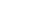xElement (mathematics)Encyclopedia
In mathematics
Mathematics
Mathematics is the study of quantity, space, structure, and change. Mathematicians seek out patterns and formulate new conjectures. Mathematicians resolve the truth or falsity of conjectures by mathematical proofs, which are arguments sufficient to convince other mathematicians of their validity...

, an element or member of a set is any one of the distinct objects that make up that set.

## Sets

Writing A = {1, 2, 3, 4 },means that the elements of the set A are the numbers 1, 2, 3 and 4. Sets of elements of A, for example {1, 2}, are subset
Subset
In mathematics, especially in set theory, a set A is a subset of a set B if A is "contained" inside B. A and B may coincide. The relationship of one set being a subset of another is called inclusion or sometimes containment...

s of A.

Sets can themselves be elements. For example consider the set B = {1, 2, {3, 4}}. The elements of B are not 1, 2, 3, and 4. Rather, there are only three elements of B, namely the numbers 1 and 2, and the set {3, 4}.

The elements of a set can be anything. For example, C = { red, green, blue }, is the set whose elements are the colors red, green and blue.

## Notation and terminology

The relation
Relation (mathematics)
In set theory and logic, a relation is a property that assigns truth values to k-tuples of individuals. Typically, the property describes a possible connection between the components of a k-tuple...

"is an element of", also called set membership, is denoted by ∈. Writingmeans that "x is an element of A". Equivalent expressions are "x is a member of A", "x belongs to A", "is in A" and "x lies in A". The expressions "A includes x" and "A contains x" are also used to mean set membership, however some authors use them to mean instead "x is a subset
Subset
In mathematics, especially in set theory, a set A is a subset of a set B if A is "contained" inside B. A and B may coincide. The relationship of one set being a subset of another is called inclusion or sometimes containment...

of A". Logician George Boolos
George Boolos
George Stephen Boolos was a philosopher and a mathematical logician who taught at the Massachusetts Institute of Technology.- Life :...

strongly urged that "contains" be used for membership only and "includes" for the subset relation only.

The LaTeX
LaTeX
LaTeX is a document markup language and document preparation system for the TeX typesetting program. Within the typesetting system, its name is styled as . The term LaTeX refers only to the language in which documents are written, not to the editor used to write those documents. In order to...

command for this symbol is "\in".

The negation
Negation
In logic and mathematics, negation, also called logical complement, is an operation on propositions, truth values, or semantic values more generally. Intuitively, the negation of a proposition is true when that proposition is false, and vice versa. In classical logic negation is normally identified...

of set membership is denoted by ∉.

## Cardinality of sets

The number of elements in a particular set is a property known as cardinality, informally this is the size of a set. In the above examples the cardinality of the set A is 4, while the cardinality of either of the sets B and C is 3. An infinite set is a set with an infinite number of elements, while a finite set is a set with a finite number of elements. The above examples are examples of finite sets. An example of an infinite set is the set of natural numbers, N = { 1, 2, 3, 4, ... }.

## Examples

Using the sets defined above:
• 2 ∈ A
• {3,4} ∈ B
• {3,4} is a member of B
• Yellow ∉ C
• The cardinality of D = { 2, 4,  8, 10, 12 } is finite and equal to 5.
• The cardinality of P = { 2, 3, 5, 7, 11, 13, ...} (the prime numbers) is infinite (this was proven by Euclid
Euclid
Euclid , fl. 300 BC, also known as Euclid of Alexandria, was a Greek mathematician, often referred to as the "Father of Geometry". He was active in Alexandria during the reign of Ptolemy I...

).